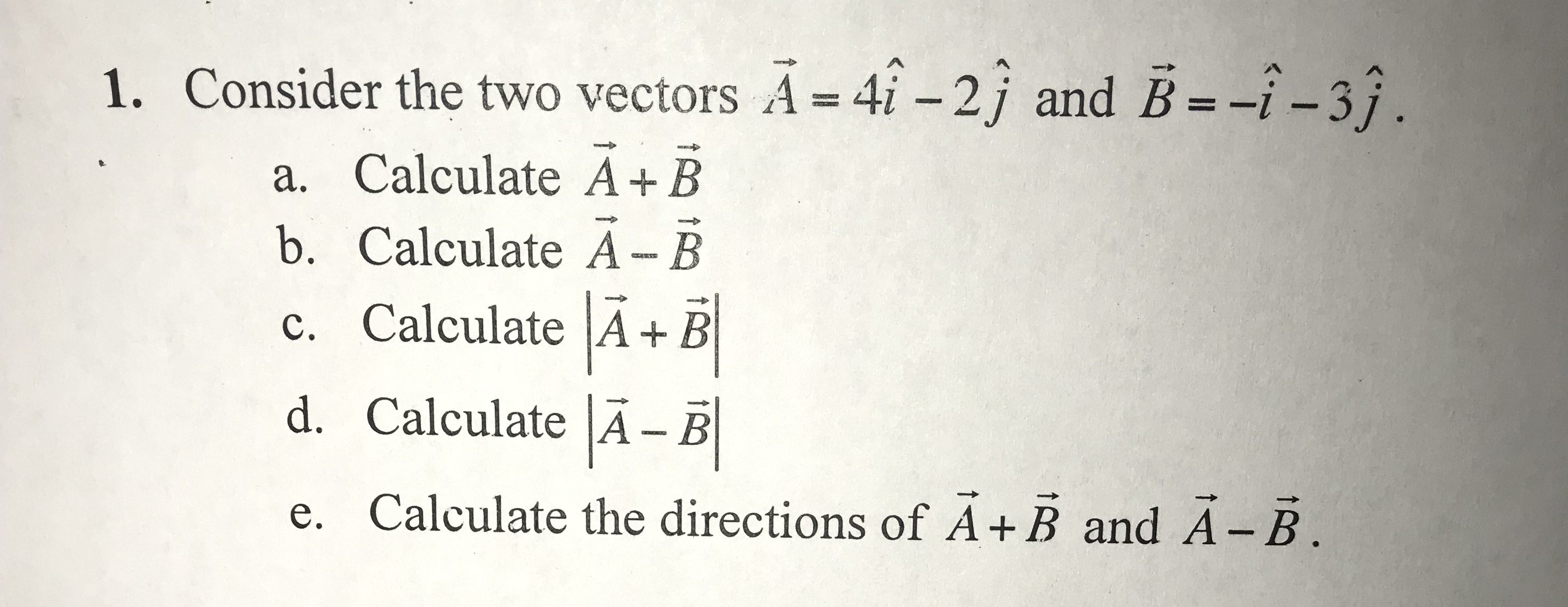# 1.Consider the two vectors A 4i -2j and B--i-3j.a. Calculate A+ Bb. Calculate A- Bc. Calculate AEd. Calculate A- Be. Calculate the directions of A+B and A - B

Question
50 views

I need help with this sample question. Thank you.help_outlineImage Transcriptionclose1. Consider the two vectors A 4i -2j and B--i-3j. a. Calculate A+ B b. Calculate A- B c. Calculate AE d. Calculate A- B e. Calculate the directions of A+B and A - B fullscreen
check_circle

Step 1

The sum of two vectors is obtained by adding the coefficients of corresponding unit vectors i , j , and k.

The sum of two vectors A, and B is given below.

Step 2

The difference of two vectors is obtained by subtracting coefficients of corresponding unit vectors i , j, and k.

The difference of two vectors A, and B is given below.

Step 3

The magnitude of a vector is obtained by taken the square root of sum of the squares of com...

### Want to see the full answer?

See Solution

#### Want to see this answer and more?

Solutions are written by subject experts who are available 24/7. Questions are typically answered within 1 hour.*

See Solution
*Response times may vary by subject and question.
Tagged in

### Physics